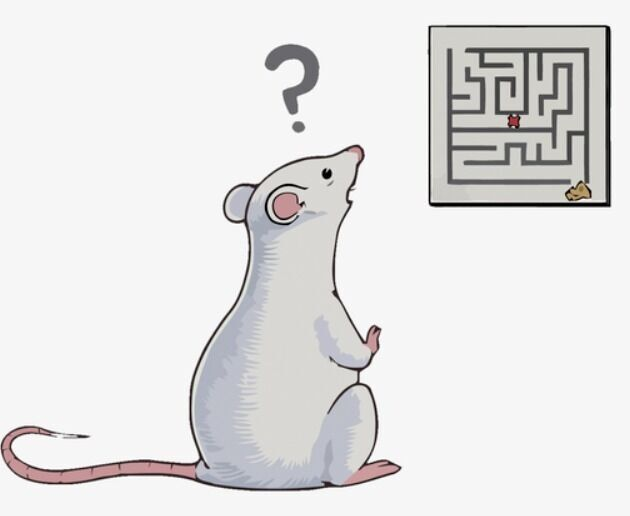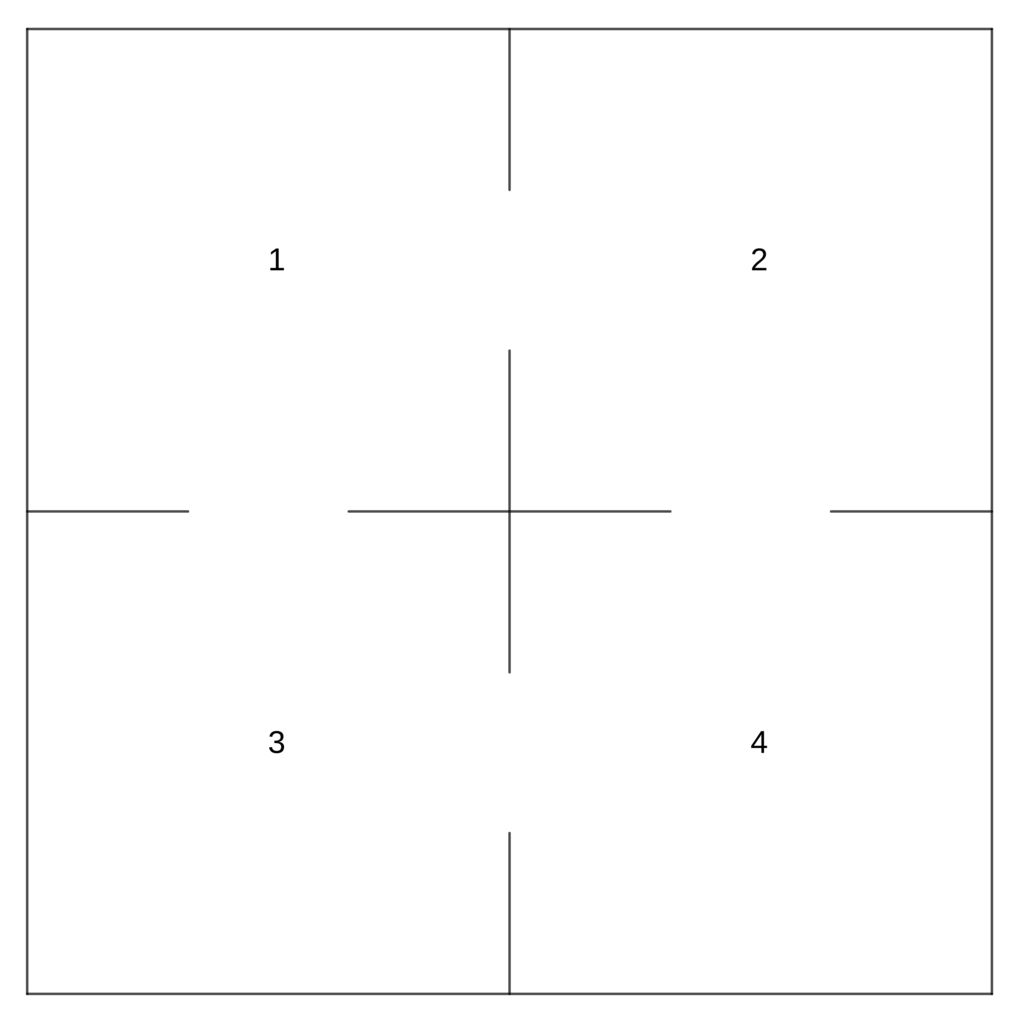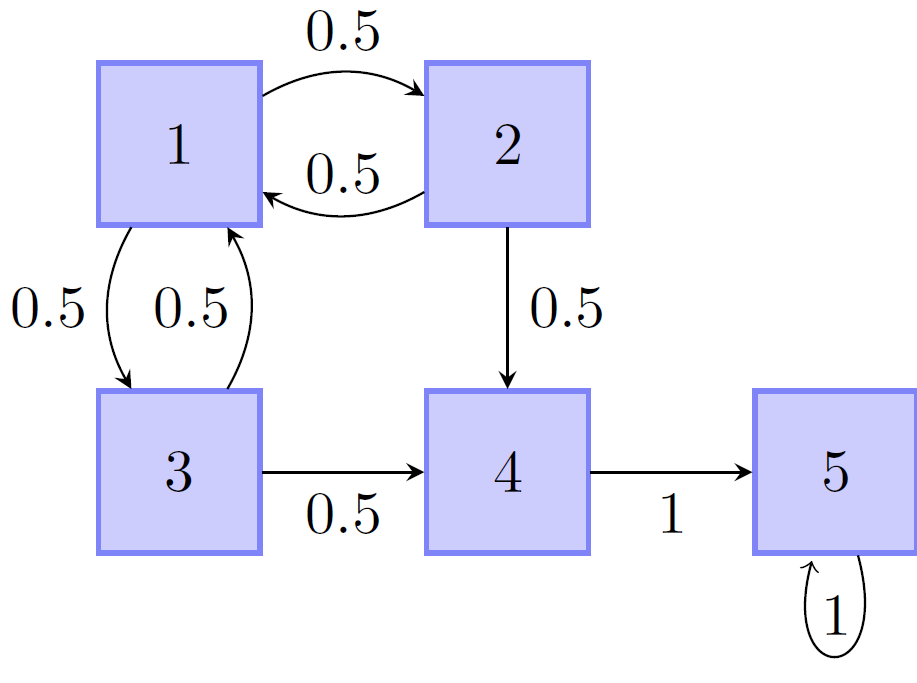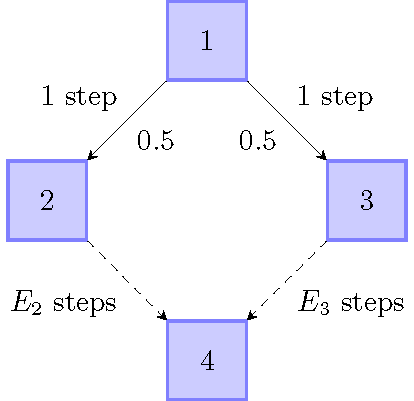# 随机过程求期望

286
9
29 名读者已经挑战成功• 若迷宫不会坍塌，则老鼠平均需要经过几分钟才能逃出迷宫？

### 问题回顾表示经过次转移后的状态向量(处于各个状态的概率所组成的向量)，之前我们得到### 随机变量及其期望

1. 用数值来表达随机试验结果的变量. 详见维基百科

2. 随机变量取值的加权平均，权重为该取值的概率. 详见维基百科

### 状态转移矩阵的修正表示的是经过次转移，老鼠落在4号格子(已逃出)的概率.### 无穷数列的求和

#!/usr/bin/env python3

import numpy as np
n = 15  # 计算到n次转移后的情况
i = 0  # 当前进行到i次转移
x = np.array([
,
,
,
,
])  # 当前的状态向量
P = np.array([
[0, 0.5, 0.5, 0, 0],
[0.5, 0, 0, 0, 0],
[0.5, 0, 0, 0, 0],
[0, 0.5, 0.5, 0, 0],
[0, 0, 0, 1, 1]
])  # 转移矩阵
while i < n:
x = P.dot(x)  # x=P*x
i = i+1
print('P(x='+str(i)+'): '+str(x))  # 输出P(x=i)\

P(x=1): [0.]
P(x=2): [0.5]
P(x=3): [0.]
P(x=4): [0.25]
P(x=5): [0.]
P(x=6): [0.125]
P(x=7): [0.]
P(x=8): [0.0625]
P(x=9): [0.]
P(x=10): [0.03125]
P(x=11): [0.]
P(x=12): [0.015625]
P(x=13): [0.]
P(x=14): [0.0078125]
P(x=15): [0.]

1. 事实上我们可以计算转移矩阵的次方，即得到各元素关于的表达式. 其计算方法请参考任一本《线性代数》的教材.1. 对于分子分母极限都为零或都为无穷大时，可以分别求导再求极限. 详见维基百科

### 蒙特卡罗统计模拟法

1. 一种借助计算机生成随机数来进行多次实验，根据结果统计来估计理论值的方法, 详见维基百科

  #!/usr/bin/env python3
import numpy as np
from random import choices
from statistics import mean

class State(object):

def __init__(self,state_id):
self.transition_matrix = np.array([
[0, 0.5, 0.5, 0],
[0.5, 0, 0, 0],
[0.5, 0, 0, 0],
[0, 0.5, 0.5, 1],
])  # 转移矩阵
self.state_id = state_id  # 状态ID： 状态1的ID为0

def move(self):
candidates = range(0, 4)  # 转移目标
weights = self.transition_matrix[:, self.state_id]  # 转移概率
result = choices(candidates, weights)  # 产生随机转移结果
return State(result)

if __name__ == '__main__':
e = 100000  # 试验次数
r = []  # 试验结果
for i in range(0,e):
cur_state = State(0)  # 初始状态: 状态1
count = 0  # 转移次数
while cur_state.state_id != 3:
cur_state = cur_state.move()
count += 1
r.append(count)  # 记录结果
print(mean(r))  # 输出均值

    4.0062

### 更简洁、一般的解法## 参考文献

 David C. Lay & Steven R. Lay & Judi J. McDonald. Linear Algebra and Its Applications. Pearson Higher Ed.